# Present Simple vs. Present Continuous ESL Activities, Worksheets and Games

## Find Someone Who...

### ESL Present Simple vs. Present Continuous Activity - Writing, Listening & Speaking - Elementary (A1-A2) - 35 minutes

This free present simple vs. present continuous 'Find someone who' activity helps to illustrate to elementary students how the present simple and present continuous tense are different and how to ask and answer questions using each tense. Give each student a copy of the worksheet. Working together, the students prepare the present simple and present continuous yes/no questions together with possible follow-up questions for the items on the worksheet. Afterwards, review the questions together as a class. Students then go around the class and ask each other the present simple and present continuous yes/no questions from the worksheet. When a student finds someone who answers 'Yes, I do' or 'Yes, I am' to a question, the student writes down their name and asks a Wh follow-up question to gain more information, noting down the answer in the last column. When everyone has finished, students give feedback to the rest of the class on what they found out by making present simple and present continuous statements.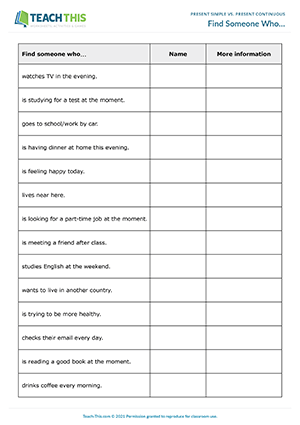## Which tense?

### ESL Present Simple vs. Present Continuous Activity - Writing, Listening & Speaking - Elementary (A1-A2) - 30 minutes

This present simple vs. present continuous activity helps elementary students practice forming and answering questions in the present simple and present continuous tense. Divide the students into two groups (A and B) and give each student a corresponding worksheet. Working alone or in pairs, the students complete the questions on the worksheet in the present simple or present continuous using the verbs in brackets. When the students have finished, check the questions with each group. After that, the students pair up with someone from the other group. The students then take it in turns to ask and answer the questions with their partner, paying close attention to the tense of each question. Afterwards, the students share what they found out about their partner with the class.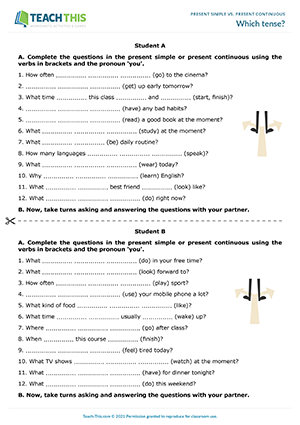Interactive Version - In this present simple vs. present continuous breakout room activity, students complete present simple and present continuous questions and then practice asking and answering the questions with a partner.

## An Unusual Monday

### ESL Present Simple vs. Present Continuous Worksheet - Reading & Writing Activity - Pre-intermediate (A2) - 30 minutes

Here is a printable story-based worksheet to help students practice the present simple vs. the present continuous. Give each student a copy of the two-page worksheet. Students begin by reading the first part of the story and then answering questions about it using the present simple. Students then read the next part of the story and write present continuous questions for a set of answers. Next, students complete the following part of the story with the present simple or present continuous form of the verbs in brackets. In the last exercise, students put words in the correct order to complete the story.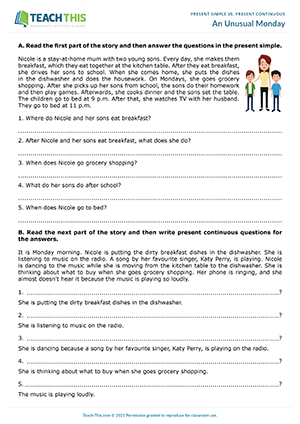Interactive Version - In the present simple vs. present continuous interactive worksheet, students complete a range of exercises to practice the present simple and present continuous tense together.

## Greetings from Italy!

### ESL Present Simple vs. Present Continuous Activity - Reading and Writing - Pre-intermediate (A2) - 35 minutes

In this present simple vs. present continuous activity, students write and then ask present simple and present continuous questions in order to complete missing information in an email. Divide the students into two groups (A and B) and give each student a corresponding worksheet. Working together, the students read the email on their worksheet and write the present simple and present continuous questions they need to ask in order to complete the missing information. When the students have finished, check the questions with each group. Next, the students pair up with someone from the other group. The students then take it in turns to ask and answer the questions they have prepared. The students write the answers in the spaces in the email. When the students have finished, they check their answers by comparing worksheets.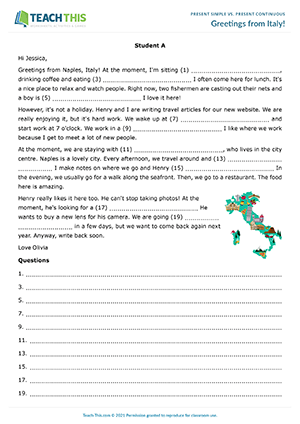Interactive Version - In this present simple and present continuous breakout room activity, pairs of students complete missing information in an email by writing and then asking present simple and present continuous questions.

## Present Simple and Continuous Question Time

### ESL Present Simple vs. Present Continuous Activity - Writing, Listening & Speaking - Pre-intermediate (A2) - 30 minutes

In this free present simple vs. present continuous activity, students write questions on various topics using both tenses. The students then ask and answer the questions with a partner. Give each pair of students a set of topic cards. Working together, the students look at the prompts on each card and write one question in the present simple and one in the present continuous. When the students have finished, check the questions with the class. The students then take it in turns to ask and answer the questions with their partner. Afterwards, the students share what they found out about their partner with the class.## Present Simple vs Present Continuous Review

### ESL Present Simple vs. Present Continuous Worksheet - Reading & Writing Activity - Pre-intermediate (A2) - 30 minutes

In this grammar review worksheet, students revise the present simple and present continuous tense. Give each student a copy of the two-page worksheet. Students begin by using phrases to write grammar rules for the present simple and the present continuous. Next, students look at a set of time expressions and decide which ones are used with the present simple and which are used with the present continuous. Students then move on to answer questions in the present simple or present continuous, according to the time expression used in each question. After that, students rewrite sentences, correcting the mistakes. In the last exercise, students complete sentences with verbs in their present simple or present continuous form.Interactive Version - In this grammar review interactive worksheet, students revise the present simple and the present continuous.

## Guess the Sentence

### ESL Present Simple vs. Present Continuous Game - Listening & Speaking Activity - Intermediate (A2-B1) - 30 minutes

In this entertaining present simple vs. present continuous activity, students play a guessing game where they describe present simple or present continuous sentences for other students to guess. Give each group of three or four a set of sentence cards, which they shuffle and place face down in a pile on the desk. The students then take it in turns to pick up a card and describe the sentence on the card using other present simple or present continuous phrases. Students can use the verb on the card but they are not allowed to say the sentence or clusters of words within the sentence. For example, if the card read 'I get up late on Sunday', the student might say 'Monday to Saturday, I get up at 6 o'clock in the morning. The other day, I get up at 11 o'clock in the morning. I like to sleep in on that day'. The other group members listen and then try to guess the sentence. The first group member to correctly guess the sentence wins and keeps the card. The student with the most cards at the end of the game is the winner.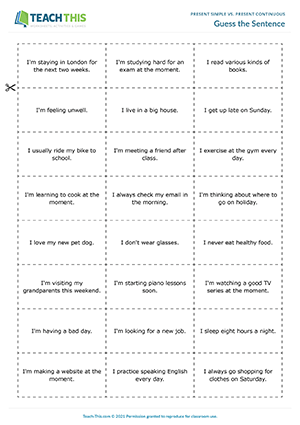## An Interview with Elon Musk

### ESL Present Simple vs. Present Continuous Activity - Writing, Listening & Speaking - Intermediate (B1) - 20 minutes

In this intriguing present simple vs. present continuous speaking activity, students take part in a business interview to practice asking and answering questions in the present simple and present continuous. Divide the students into pairs. Give one student a journalist worksheet and the other an Elon Musk worksheet. The journalist's task is to interview Elon Musk to find out about his work routine, current projects, plans and goals, and make notes on his answers. The student who is Elon Musk uses the information on the worksheet to answer the journalist's questions. The students prepare what they are going to say, paying close attention to which parts of the interview will be in the present simple and which parts will be in the present continuous. The students then role-play the interview in their pairs. When the pairs have finished, they report back to the class on their interviews. As an extension, the pairs write a short news article in the present simple and present continuous based on the interview.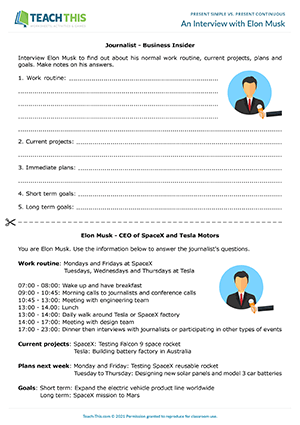## Create a Company

### ESL Present Simple vs. Present Continuous Activity - Writing, Listening & Speaking - Intermediate (B1) - 45 minutes

In this present simple vs. present continuous activity, students create a company by completing and answering questions in the present simple and present continuous. Give each student a copy of the worksheet. The students begin by completing questions on the worksheet in the present simple or present continuous. When the students have finished, check the answers with the class. Next, divide the students into small groups. The students then create a company by inventing answers to the questions on the worksheet. In their groups, the students discuss each question in turn and write an answer in sentence form using the present simple or present continuous. When the groups have finished, they take it in turns to present their company using the information they created. After each presentation, give feedback and invite questions from the class.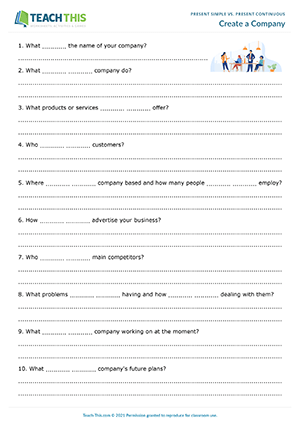Interactive Version - In this breakout room activity, groups of students create a company by completing and answering present simple and present continuous questions.

0
0
0
s2sdefault